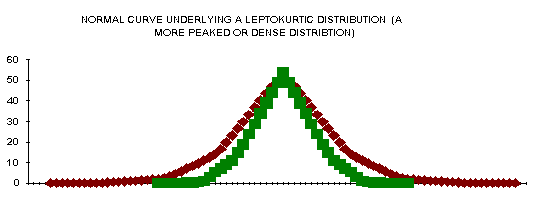previous lesson

Graphic and Tabular Presentation of Data

TABULAR PRESENTATION OF DATA

Raw Data

 Ungrouped Data Grouped Data Ages Ages f or Ages f 25 18 2 18-19 5 45 19 3 20-21 23 22 20 11 22-23 22 23 21 12 24-25 6 46 22 15 26-27 4 84 23 7 28-29 etc. 12 24 4 30-31 2 25 2 32-33 34 26 3 34-35 15 27 1 etc.

* note the difference is width of category; 1 versus 2 years

1. How to group data: some guidelines
• Number of categories should be great enough so accuracy is not sacrificed too much.
Example of too much:

 Ages f 18-31 72 32-45 45

• Number of categories should be small enough to avoid vacant categories or great fluctuations between categories.
• All categories should be equal in width (this is especially important).
1. 2. Almost every measurement is rounded off, such as height ("5 foot 9 inches" instead of "5 foot 8.89 inches"). One major exception to this is age. Age is looked at two ways:

 Age to last Birthday Age to nearest Birthday Age limits True limits midpoint Age limits true limits midpoint 18-19 18.00-19.999 19.00 18-19 17.50-19.4999 18.5 20-21 20.00-21.999 21.00 20-21 19.50-21.4999 20.5 etc.

m (for continuous variables) =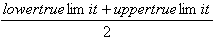m (for discrete variables) =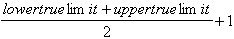*midpoint: abbreviated : "m"

*discrete variable: the variable's unit of measure cannot be divided infinitely

*continuous variable: the variable's unit of measure that can be divided infinitely.

GRAPHING DATA

1. Histogram -- for interval data
• width of rectangle = width of category
• rectangles are centered over the midpoints of the category
• rectangles are connected to each other to indicate their continuous nature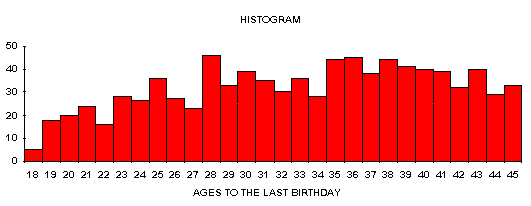B. Bar graph -- for nominal data (or ordinal)

• order is irrelevant unless using ordinal data
• bars can be of any width, but are all equal in width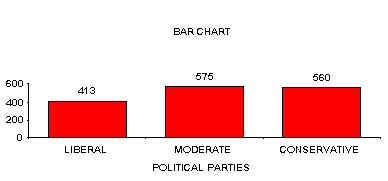SOURCE: GSS91 SURVEY SUBSAMPLE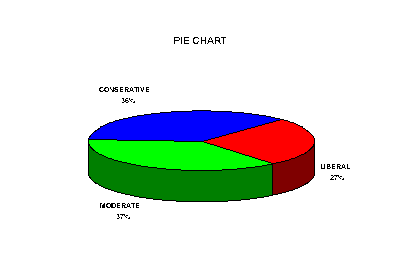C. Pie Chart -- for nominal data (or ordinal)

• slices are proportional in width
• order is irrelevant unless using ordinal data

SOURCE: GSS91 SURVEY SUBSAMPLE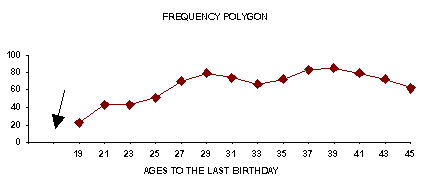*Double hatch marks indicate interruption in the consistently equal intervals. In this case the earliest age in the sample was 15. The double hatch marks indicates that the range 0-9.99 was skipped in the polygon.

D. Frequency polygon -- for interval data

• often used for scientific purposes
• need midpoints; plot these
• connect the dots

TYPES OF DISTRIBUTION

A. unimodal = one peak (mode)

• "normal curve," "Bell shape distribution"
• symmetrical (right side = left)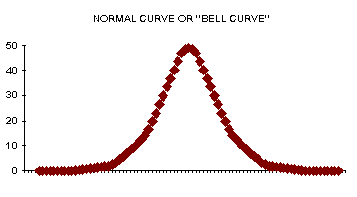B. bimodal = 2 modes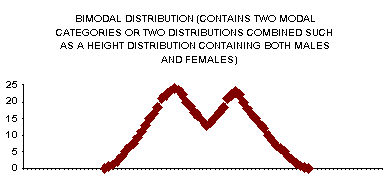C. Skewed distributions are unimodal distributions which are not symmetrical. A positively skewed distribution will have a mean that is greater in value than its median. Its tail will fall on the side of the larger values. A negatively skewed distribution will have a median that is greater in value than it's mean. Its tail will fall on the side of the smaller values. A normal curve skew measures 0, while a positive skew measure is a positive value and the negative skew measure is a negative value.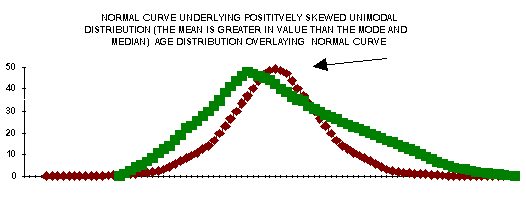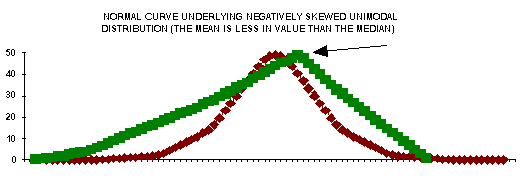D. Degree of kurtosis -- more dense or peaked distributions than the Bell curve are called leptokurtic. Flatter distributions than the Bell curve are called platykurtic. A normal kurtosis measures 0. A positive value of kurtosis describes a leptokurtic distribution, while a negative value of kurtosis describes a platykurtic distribution.